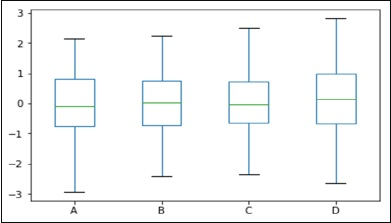## Pandas Built in Data Visualization exercise and solution:

Want to learn Pandas Built in Data Visualization?
Hello friendes, welcome to my blog post Pandas Built in Data Visualization exercise and solution. In the previous blog post we see what is pandas built in data visualization so it’s time to solve exercise, don’t worry solution also present in drive file. Be for start the exercise we will see some basics of pandas data visualization. Let’s start:
Everything you need to know about Pandas

Pandas is open source data structures and data analysis tool for python programming.  Python is the most popular data science language to learn in 2020. The name Pandas is derived from the words Panel Data — an Econometrics from Multidimensional data.This tutorial will offer a beginner guide into how to get around with Pandas for data wrangling and visualizations.

### Import Pandas

We start by importing pandas and aliasing, It as pd to give us a short form to use in our analysis.
import pandas as pdfig 01) Pandas Built in Data Visualization exercise and solution

Pandas allows you to import files in various formatThe most popular format is CSV.
The first step is to assign the files you are going to load to a variable in order to be able to manipulate the data frame later in your analyses. A data frame is basically the representation of the row and column in your dataset.

For A csv file

For An Excel File
SOME BASIC EXAMPLE:
BOX PLOT

`import pandas as pdimport numpy as npdf = pd.DataFrame(np.random.rand(10, 4), columns=['A', 'B', 'C', 'D'])df.plot.box()`
Its output is as follows −